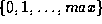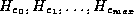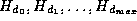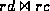Next: Three-Way Join Up: Join Strategies Previous: Use of an Index

Hash Join

1. Sometimes it may be useful to construct a ``use once only'' hash structure to assist in the computation of a single join.
• We use a hash function h to hash tuples of both relations on the basis of join attributes.
• The resulting buckets, pointing to tuples in the relations, limit the number of pairs of tuples that must be compared.
• If d is a tuple in deposit and c is a tuple in customer, then d and c must be compared only if h(d) = h(c).
• The comparison must still be done as it is possible that d and c have different customer names that hash to the same value.
• As before, we need our hash function to hash randomly and uniformly.
2. We will now estimate the cost of a hash-join.

• Assume that h is a hash function mapping cname values to.
•denote buckets of pointers to customer tuples, each initially empty.
•denote buckets of pointers to deposit tuples, each initially empty.
• The hash-join algorithm is shown in figure 9.4.
• The first two loops assign pointers to the hash buckets, requiring a complete reading of both relations.
• This requires 510 block accesses if we assume that both relations are stored together physically (i.e. not clustered with other relations).
• As buckets contain only pointers, we assume they fit in main memory, so no disk accesses are required to read the buckets.
• Final loop of the algorithm iterates over the range of hash function h.
• Assume i is a particular value in the range of h.
• The tuples rd and rc are assembled, from the pointers, where rd is the set of deposit tuples that hash to bucket i, and rc is the set of customer tuples that hash to bucket i.
• Thenis calculated.
• This join is done using simple iteration, since we expect rd and rc to be small enough to fit in main memory.
• Since a tuple hashes to exactly one bucket, each tuple is read only once by the final outer loop.
• Earlier printings of the text say this requires another 510 block accesses, for a total of 1020. This is wrong!
• If there are 10,000 deposit tuples and 200 customer tuples, then reading every tuple once could take 10,200 accesses, worst-case.
• Total is then 510 block accesses to create the buckets, plus 10,200 to assemble the buckets' records, giving 10,710.
• Exercise: calculate the cost by other methods. What have we gained, and at what cost?
3. If the query optimizer chooses to do a hash-join, the hash function must be chosen so that
• The range is large enough to ensure that buckets have a small number of pointers, so that rd and rc fit in main memory.
• The range is not so large that many buckets are empty and the algorithm processes many empty buckets.Next: Three-Way Join Up: Join Strategies Previous: Use of an Index

Osmar Zaiane
Sun Jul 26 17:45:14 PDT 1998